Note: this document will print in an appropriately modified format (5 pages)

1  Which base gets the proton?

Will acid HA react with base B? We stated above that the outcome of any acid-base reaction depends on how well two different bases B1 and B2 can compete in the tug-of-war for the proton

B1H+→ B2(1-1)

The proton will always go to the stronger base. Some insight into this can be had by thinking of the proton as having different potential energies when it is bound to different acceptors. We can draw a useful analogy with the electrons in an atom, which, you will recall, will always fall into the lowest-potential energy orbitals available, filling them from the bottom up. In a similar way, protons will "fall" into the lowest-energy empty spots (bases) they can find.

### Proton energy, sources and sinks

In the illustration on the right, the ion A is the conjugate base of the acid HA, and thus corresponds to one of the bases in Eq. 1 above.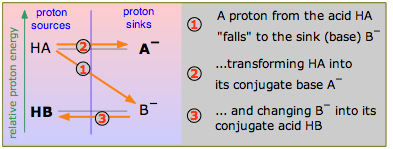Consider the scheme shown here, which depicts two hypothetical acid-base conjugate pairs. Take careful note of the labeling of this diagram: the acids HA and HB are proton sources and the conjugate bases A and B are proton sinks. This "source-sink" terminology is synonymous with the "donor-acceptor" language that Brønsted taught us, but it also carries an implication about the relative energies of the proton as it exists in the two molecules HA and HB. If, as is indicated here, the proton is at a higher "potential energy" when it is in the form of HA than in HB, the reaction HA + B → HB + A will be favored compared to the reverse process HB + A → HA + B, which would require elevating the proton up to the A level. In this example, HA is the stronger acid because its proton can fall to a lower potential energy when it joins with B to form HB.

We will refer to diagrams such as the one above as "proton-energy diagrams", which is not quite correct, but we don't want to get into thermodynamics at this point. (If you already know something about chemical thermodynamics, we are really referring to Gibbs free energy.)

It follows, then, that if we can arrange all the common acid-base conjugate pairs on this kind of a scale, we can predict the direction of any simple acid-base reaction without resorting to numbers. This will be illustrated further on, but in order to keep things simple, let's look at a few proton-energy diagrams that illustrate some of the acid-base chemistry that we discussed in the preceding section.

### How do we define "strong" and "weak" acids?

The hydronium ion is the dividing line; a strong acid, you will recall, is one whose conjugate base A loses out to the "stronger" base H2O in the competition for the proton:

A H+→ H2O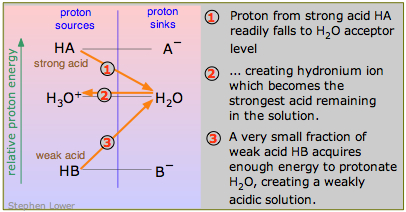An acid that is a stronger proton donor than hydronium ion is a strong acid; if it is a weaker proton donor than H3O+, it is by definition a weak acid. This is seen most clearly in the diagram here, which contrasts the strong acid HA with the weak acid HB. HB "dissociates" to only a tiny extent because it is energetically unfavorable to promote its proton up to the H2O-H3O+ level (process 3 in the diagram).

#### Strong acids and leveling

A strong acid, you will recall, is one whose conjugate base A loses out to the "stronger" base H2O in the competition for the proton:

A H+→ H2O

Because the reaction

HA + H2O → A+ H3O+

for any strong acid HA is virtually complete, all strong acids appear to be equally strong in water (the leveling effect.)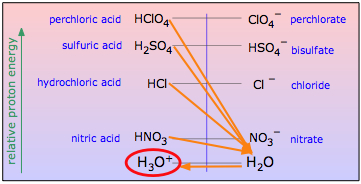From the proton-energy standpoint, a strong acid is one in which the energy of the proton is substantially greater when attached to the anion A than when it is attached to H2O. Adding a strong acid HA to water will put it in contact with a huge proton sink that drains off the protons from any such acid, leaving the conjugate base A along with hydronium ion, the strongest acid that can exist in water.

The weakest acid and the strongest base

For a very long time, chemists had regarded methane, CH4, as the weakest acid, making the methide ion CH3 (which is also the simplest carbanion) the strongest base. Methane still holds its position as the weakest acid, but in 2008, the ion LiO was found to be an even stronger base than CH4. Because both of these bases are observable only in the gas phase, these facts have little obvious import on aqueous-solution chemistry.

### Autoprotolysis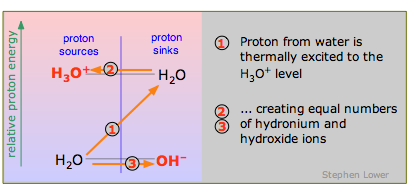Because water is amphiprotic, one H2O molecule can donate a proton to another, as explained above. In this case the proton has to acquire considerable energy to make the jump (1) from the H2O-OH level to the H3O+-H2O level, so the reaction

2 H2O → H3O++ OH

occurs only to a minute extent. Think of this as the special case of the "weakest" acid H2O reacting with the "weakest" base H2O.

### Strong bases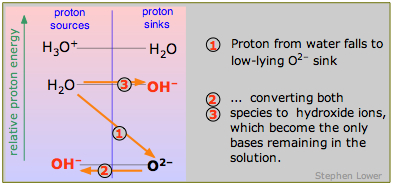Finally, what is a strong base? Just as a strong acid lies above the H3O+-H2O level, so does a strong base lie below the H2O-OH level. And for the same reason that H3O+ is the strongest acid that can exist in water, OH is the strongest base that can exist in water. The example of the oxide ion O2– is shown here. Sodium oxide Na2O is a white powder that dissolves in water to give oxide ions which immediately decompose into hydroxide ions

O2– + H2O → 2 OH

### Putting it all together, and the meaning of pH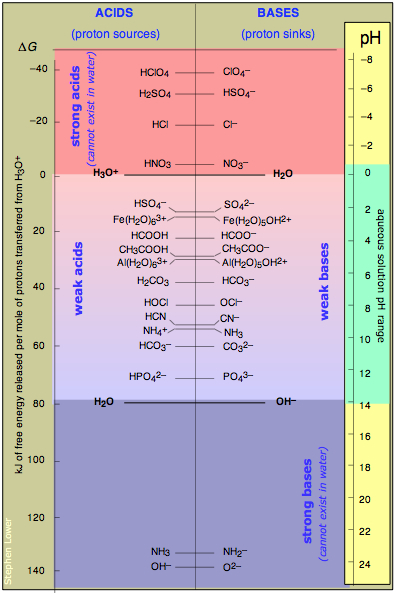This table combines common examples covering the entire range of acid-base strengths, from the strong to the very weak. The energy scale at the left gives you some idea of the relative proton-energy levels for each conjugate pair; notice that the zero is arbitrarily set to that of the H3O+-H2O pair.

Of more importance is the pH scale on the right. The pH that corresponds to any conjugate pair is the pH at which equal concentrations of that pair are in their acid and base forms. For example, acetic acid
CH3COOH is "half ionized" at a pH of 4.7. If another strong acid such as HCl is added so as to reduce the pH, the proportion of acetate ion decreases, while if sodium hydroxide is added to force the pH higher, a larger fraction of the acetic acid will be "dissociated".

This illustrates another aspect of pH: at its most fundamental level, pH is an inverse measure of the "proton intensity" in the solution. The lower the pH, the higher the proton intensity, and the greater will be the fraction of higher-energy proton levels populated— which translates to higher acid-to-conjugate base concentration ratios.

It is easy to see why acids such as H2SO4 and bases such as the amide ion NH2 cannot exist in aqueous solution; the pH would have to be at the impossible level of –6 for the former and +23 for the latter!

#### Why acids are titrated with hydroxide ion

When you titrate an acid with a base, you want virtually every molecule of the acid to react with the base. In the case of a weak acid such as hypochlorous, the reaction would be

HOCl + OH → OCl + H2O

Because the proton level in HOCl is considerably above that in H2O, titration with NaOH solution will ensure that every last proton is eaten up by the hydroxide ion. If, instead, you used ammonia NH3 as a titrant, the closeness of the two proton levels would cause the reaction to be incomplete, yielding a less distinct equivalence point. And, of course, titration with a base that is weaker then hypochlorite ion (such as sodium bicarbonate) would be hopeless.

As a practical matter, you can usually estimate that when the pH differs by more than about two units from the value that corresponds to the conjugate-pair for a monoprotic acid, the concentraton of the non-favored species will be down by a factor of around 1000.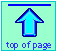Chem1 Proton donors and acceptors develops the principle that acid-base chemistryis fundamentally a competition of two bases (one of which ma be water) for the proton. It is part of the General Chemistry Virtual Textbook, a free, online reference textbook for General Chemistry by of Simon Fraser University .

This chapter covers the following topics: Understand proton-enegy diagrams, strong and weak acids, autoprotolysis. It can be accessed directly at .